Homework Help Question & Answers

# Check Your Learning Ethanol and acetic acid react and form water and ethyl acetate,the solvent responsible...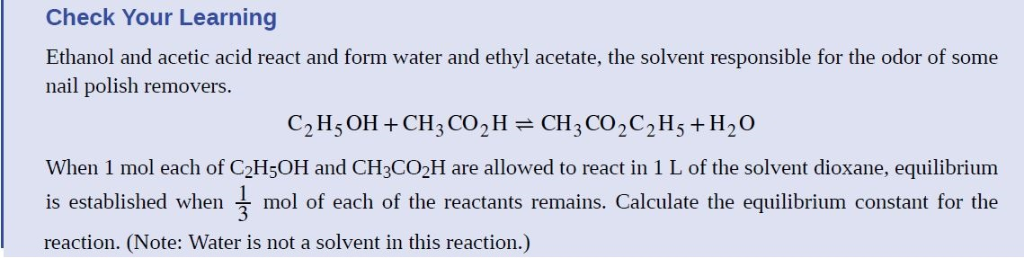Check Your Learning Ethanol and acetic acid react and form water and ethyl acetate,the solvent responsible for the odor of some nail polish removers. 2 15 When 1 mol each of C2H5OH and CH3CO2H are allowed to react in 1 L of the solvent dioxane, equilibrium is established when mol of each of the reactants remains. Calculate the equilibrium constant for the reaction. (Note: Water is not a solvent in this reaction.)

#### Homework Answers

Answer #1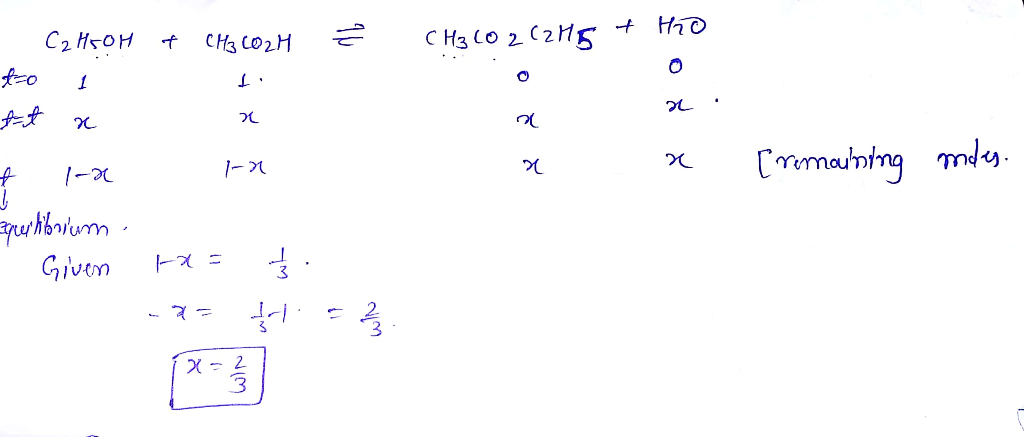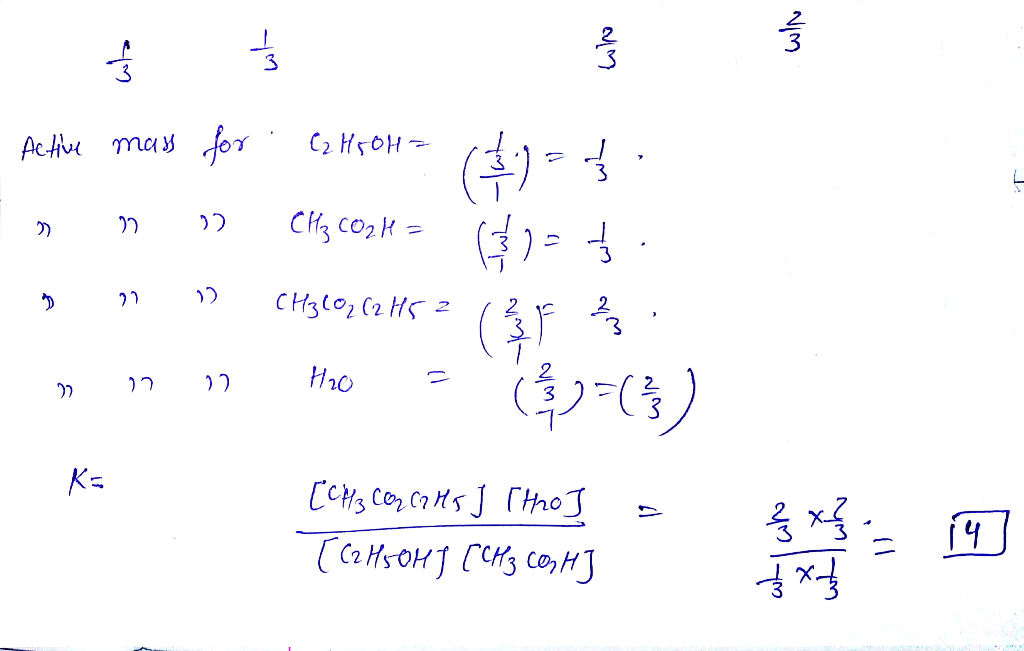*********THANK YOU *********

Know the answer?
Your Answer:

#### Post as a guest

Your Name:

What's your source?

#### Earn Coin

Coins can be redeemed for fabulous gifts.

Not the answer you're looking for? Ask your own homework help question. Our experts will answer your question WITHIN MINUTES for Free.
Similar Homework Help Questions
• ### 1.0 mol of ethanol and 1.0 mol of acetic acid are dissolved in water and kept...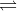1.0 mol of ethanol and 1.0 mol of acetic acid are dissolved in water and kept at 100? degrees C. The volume of the solution is 250 mL. At equilibrium, 0.25 mol of acetic acid has been consumed in producing ethyl acetate. Calculate Kc? at 100 degrees C for the reaction: C2H5OH?(aq) + CH3CO2H(aq) CH3CO2C2H5(aq) + H2O?(l)

• ### Acetic acid and ethanol react to form ethyl acetate and water, like this: HCH_3 CO_2(aq) +...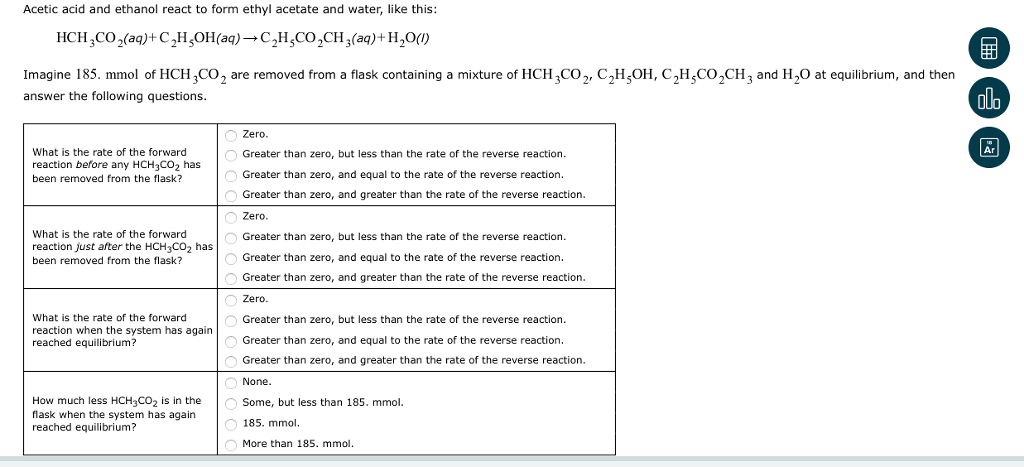Acetic acid and ethanol react to form ethyl acetate and water, like this: HCH_3 CO_2(aq) + C_2 H_5 OH(aq) rightarrow C_2 H_5 CO_2 CH_3(aq) + H_2 O(I) Imagine 185. mmol of HCH_3 CO_2 are removed from a flask containing a mixture of HCH_3 CO_2, C_2 H_5 OH, C_2 H_5 CO_2 CH_3 and H_2 O at equilibrium, and then answer the following questions. What is the rate of the forward reaction before any HCH_3 CO_2 has been removed from the flask?...

• ### Acetic acid and ethanol react to form ethyl acetate and water, like this: HCH,CO2(aq)-C2H5OH(aq) ? C2H,CO2CH3(aq)+...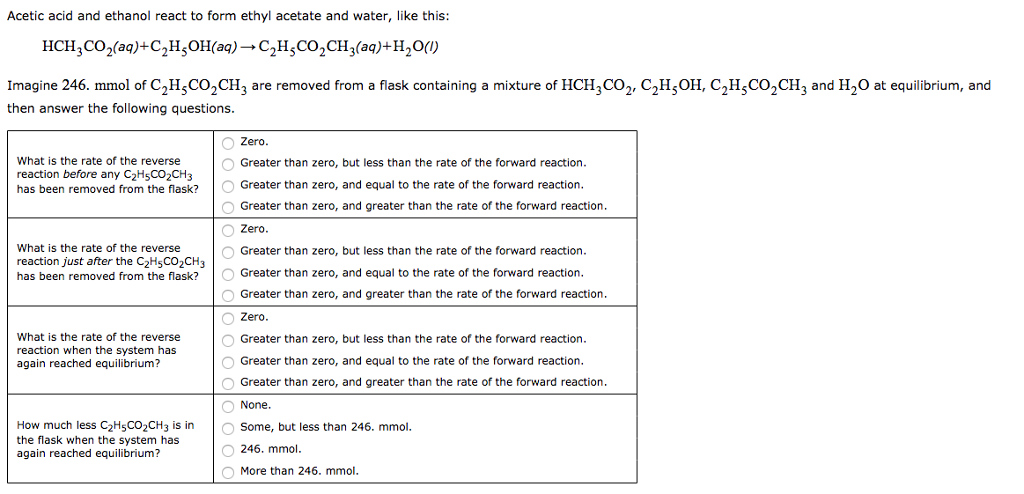Acetic acid and ethanol react to form ethyl acetate and water, like this: HCH,CO2(aq)-C2H5OH(aq) ? C2H,CO2CH3(aq)+ H2O() Imagine 246. mmol of C2HCO2CH3 are removed from a flask containing a mixture of HCH3CO2, C2H;OH, C/HCO2CH3 and H2O at equilibrium, and then answer the following questions Zero Greater than zero, but less than the rate of the forward reaction Greater than zero, and equal to the rate of the forward reaction Greater than zero, and greater than the rate of the forward...

• ### Q.1 (a) An aqueous solution of ethanol and acetic acid is heated to 100oC. Each substance...

Q.1 (a) An aqueous solution of ethanol and acetic acid is heated to 100oC. Each substance has an initial concentration of 0.810 mol L-1. At equilibrium, the acetic acid concentration is 0.748 mol L-1. Calculate the value of the equilibrium, K, for the reaction. Equation C2H5OH(aq) + CH3CO2H(aq CH3CO2C2H5(aq) + H2O(l) Initial (M) Change (M) Equilibrium (M) 0.748 (b) Propose two methods that would result in an increased concentration of CH3CO2C2H5(aq).

• ### Thank you very much in advance. Emrah Acetic acid and ethanol react to form ethyl acetate and water, like this: Imagine 53. mmol of HCH, CO, are added to a flask containing a mixture of HCH,CO...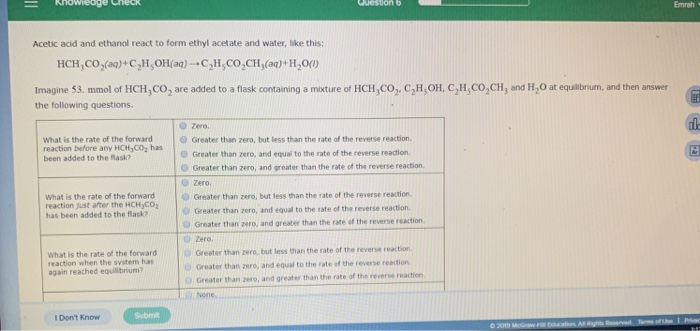Thank you very much in advance. Emrah Acetic acid and ethanol react to form ethyl acetate and water, like this: Imagine 53. mmol of HCH, CO, are added to a flask containing a mixture of HCH,CO, CH,OH, C,H,CO,CH, and H,0 at equlbnum, and then answer the following questions. O zero. What is the rate of the forward Greater than zero, but less than the rate of the reverse reaction. reaction before any HCH,002 has been added to the lask? O...

• ### 1. The reaction mixture contains isopentyl acetate, isopentyl alcohol, water, acetic acid and sulfuric acid. a)...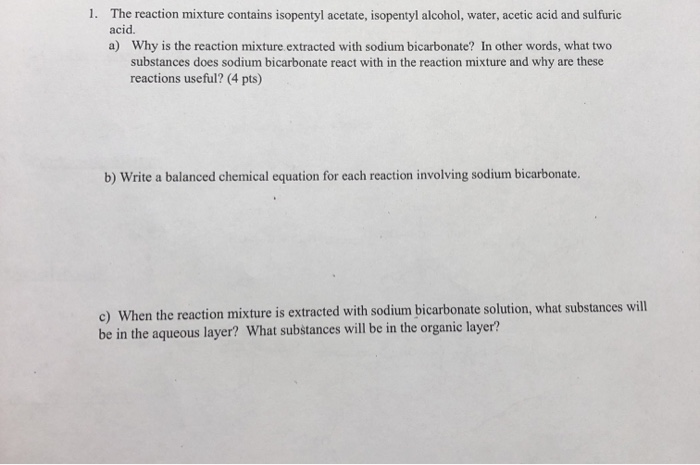1. The reaction mixture contains isopentyl acetate, isopentyl alcohol, water, acetic acid and sulfuric acid. a) Why is the reaction mixture extracted with sodium bicarbonate? In other words, what two substances does sodium bicarbonate react with in the reaction mixture and why are these reactions useful? (4 pts) b) Write a balanced chemical equation for each reaction involving sodium bicarbonate. c) When the reaction mixture is extracted with sodium bicarbonate solution, what substances will be in the aqueous layer? What...

• ### 1) Which reactant, salicylic acid or acetic anhydride, is in excess? Show your work. 2) If you we...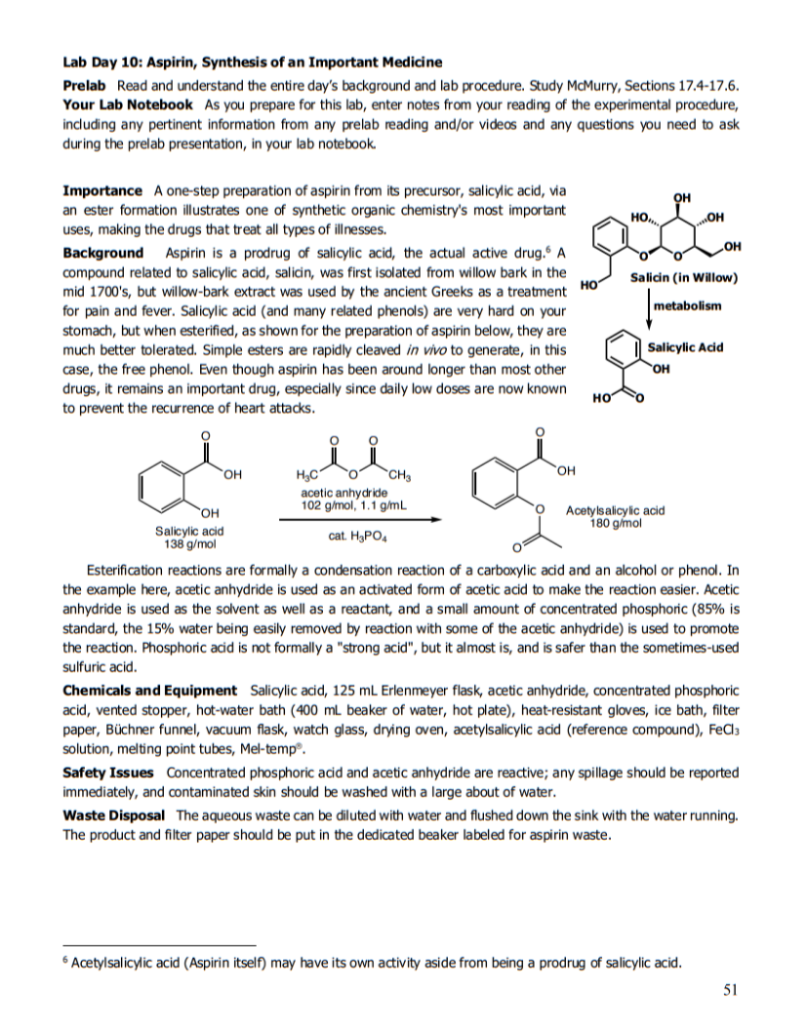1) Which reactant, salicylic acid or acetic anhydride, is in excess? Show your work. 2) If you were to use 3.17 g of starting material, how much product would you expect? Again, show your work. 3) Why is phosphoric acid added to the reaction? 4) A small amount of water is added at the end of the reaction to react with the excess acetic anhydride. Show a balanced equation for that reaction. 5) Explain the purpose of the FeCl3 test...

• ### ANSWER ALL QUESTIONS QUESTION 1 [20 MARKS] Equilibrium constant, Ke for ethanoic acid esterification (CH3COOH) with...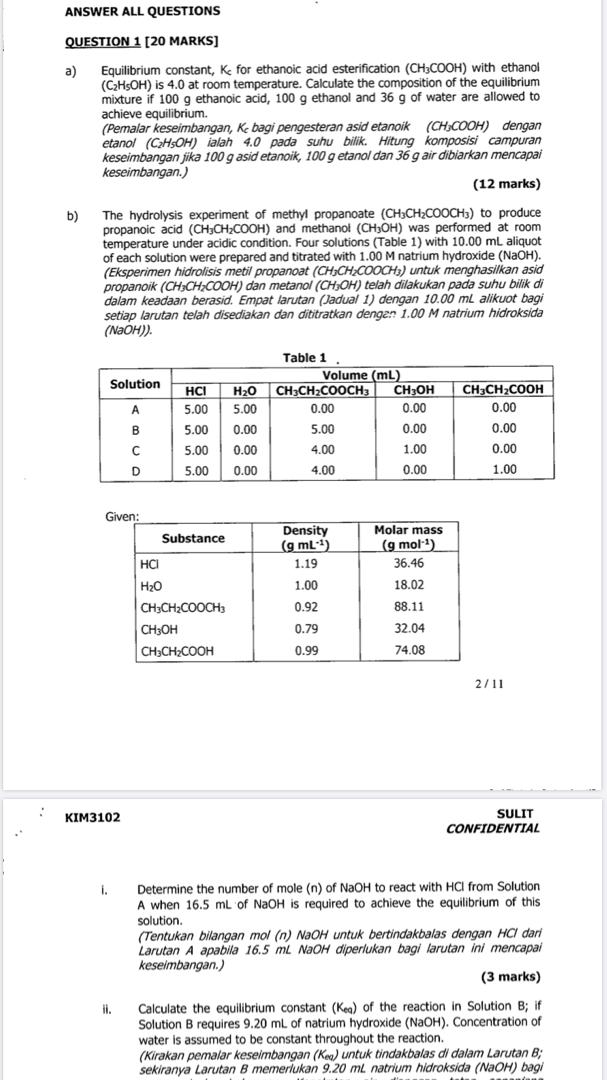ANSWER ALL QUESTIONS QUESTION 1 [20 MARKS] Equilibrium constant, Ke for ethanoic acid esterification (CH3COOH) with ethanol (C2H5OH) is 4.0 at room temperature. Calculate the composition of the equilibrium mixture if 100 g ethanoic acid, 100 g ethanol and 36 g of water are allowed to achieve equilibrium. (Pemalar keseimbangan, K bagi pengesteran asid etanoik (CH COOH) dengan etanol (CH5OH) ialah 4.0 pada suhu bilik. Hitung komposisi campuran keseimbangan jika 100 g asid etanoik, 100 g etanol dan 36 g...

• ### Please I need help on this. I'm so lost 1. Acetic acid (H)CO2H) has k, =...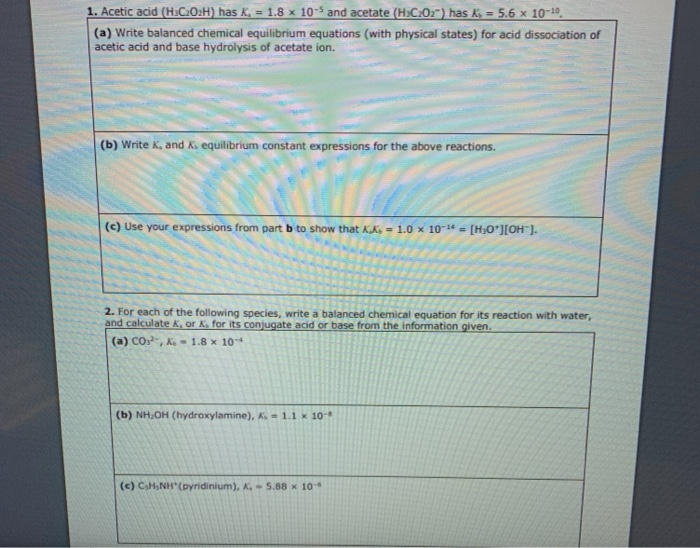Please I need help on this. I'm so lost 1. Acetic acid (H)CO2H) has k, = 1.8 x 10- and acetate (H:C:01") has ks = 5.6 x 10-10. (a) Write balanced chemical equilibrium equations (with physical states) for acid dissociation of acetic acid and base hydrolysis of acetate ion. (b) Write K, and equilibrium constant expressions for the above reactions. (c) Use your expressions from part b to show that Kx. = 1.0 x 10-* = [H,O'][OH). 2. For each...

• ### It's a weak acid strong base titration Experiment 4: Identification of an unknown acid by titration...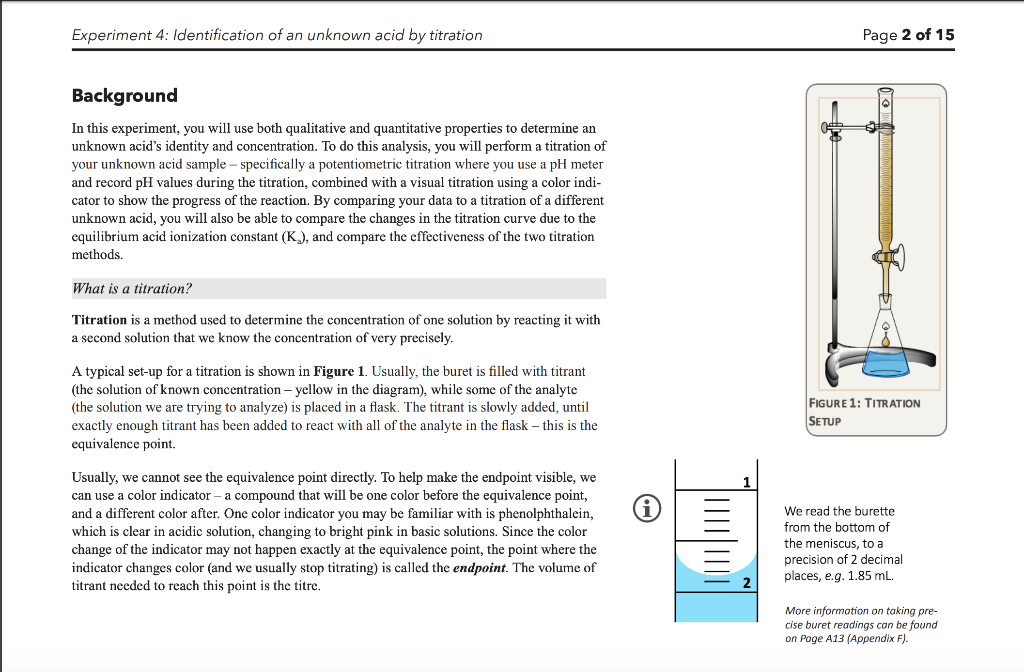It's a weak acid strong base titration Experiment 4: Identification of an unknown acid by titration Page 2 of 15 Background In this experiment, you will use both qualitative and quantitative properties to determine an unknown acid's identity and concentration. To do this analysis, you will perform a titration of your unknown acid sample-specifically a potentiometric titration where you use a pH meter and record pH values during the titration, combined with a visual titration using a color indi- cator...